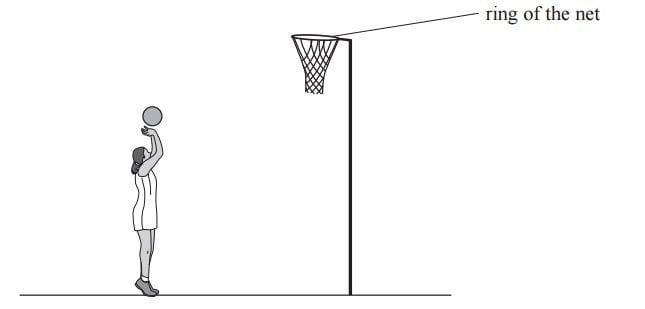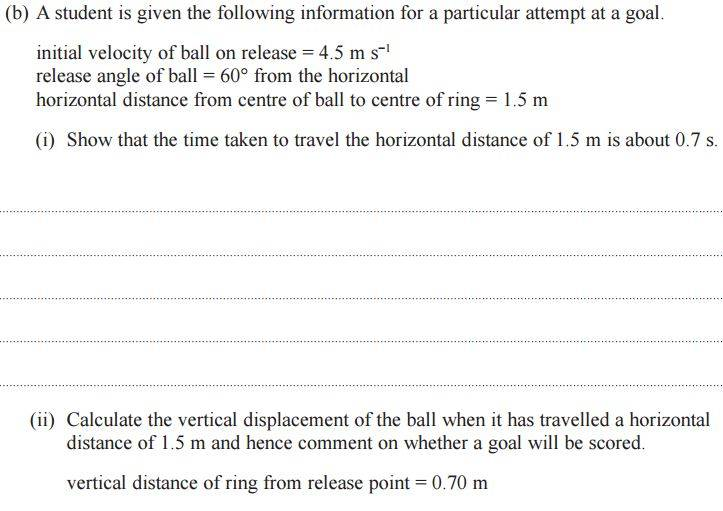# Kinematic equations for max height

## Homework Statement## The Attempt at a Solution

So (i) was easy enough and I got a time of 0.67 seconds.

For (ii), since the horizontal time is 0.67 seconds this means the TOTAL time spent in air is also 0.67 seconds, so to calculate max height we split this time in half (since the ball has to go up to reach max height and then fall down again) and use the kinematic equations to find a value of S (distance)

But they have kept the time, t, as 0.67 seconds and not 0.67/2, why is this?

gneill
Mentor
They didn't ask for the maximum height. They asked for the vertical displacement when the ball has traveled 1.5 m horizontally (i.e. at the horizontal distance of the ring).

•ravsterphysics
They didn't ask for the maximum height. They asked for the vertical displacement when the ball has traveled 1.5 m horizontally (i.e. at the horizontal distance of the ring).

But max vertical displacement occurs when time spent in air is t/2 (compared to horizontal time) and when v=0, if you take a look at this video you can see the guy did the same thing; he's working out vertical displacement using v=0 which is the same as total air time divided by 2?

gneill
Mentor
But they did not ask for maximum displacement. They asked for the displacement at a particular horizontal distance.

•ravsterphysics

## Homework Statement

View attachment 111407
View attachment 111408

## The Attempt at a Solution

So (i) was easy enough and I got a time of 0.67 seconds.

For (ii), since the horizontal time is 0.67 seconds this means the TOTAL time spent in air is also 0.67 seconds, so to calculate max height we split this time in half (since the ball has to go up to reach max height and then fall down again) and use the kinematic equations to find a value of S (distance)

But they have kept the time, t, as 0.67 seconds and not 0.67/2, why is this?

Ignoring for the moment the fact that they didn't ask you for the maximum height, your assumption that the maximum height occurs at t/2 is incorrect. That would only be true if it started and ended at the same height. If you actually did need to find the maximum height you would use the initial vertical speed and the known acceleration of gravity.

•ravsterphysics
CWatters
•